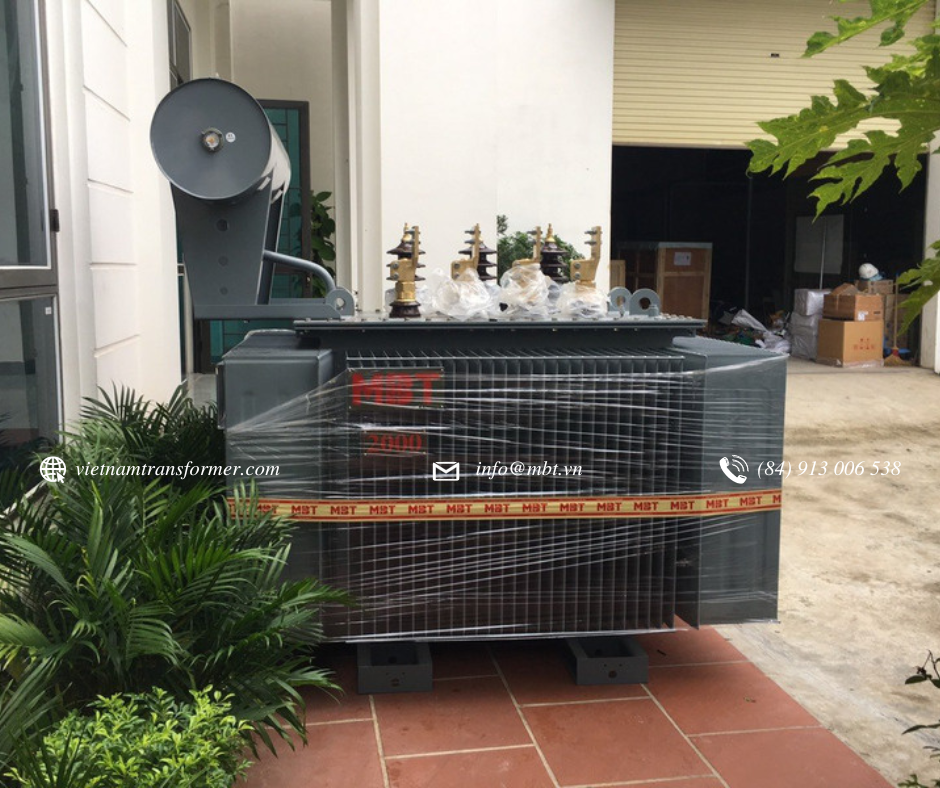# What is a Transformer Rating?

Transformers are designed based on the rated voltage and current required for the transformer’s operation. The manufacturers then describe this on the transformer’s nameplate in terms of VA (Volt-Amps) or KVA, which is referred to as the rating of a transformer. The transformer's ratings can also be the maximum current and voltage that can be safely applied to the transformer.

The rating of a transformer is calculated depending upon temperature rise, which depends on the transformer’s losses. However, Transformers are designed to limit the temperature based on the desired load, including, the hottest-spot temperature rise of a winding, the average temperature rise of a winding, and in the case of liquid-filled units, the top liquid temperature rise.

However, the Temperature rise of the transformer can be limited by using the proper cooling system. The greater the effectiveness of the cooling system, the higher the rating of the transformer (and vice versa). For a given cooling system, the rating of an electrical machine is indirectly determined by the losses present in the machine.

There are two main types of losses in a transformer:

1. Variable losses or ohmic (I2R) losses – These depend on the I
2. Constant losses or core losses – These depend on V

Hence total losses depend on V and I. Because the rating of a transformer depends upon losses and losses depend upon I and V, the rating of a transformer depends on V×I, which is also termed VI ratings.

As losses are independent of the power factor of load, the rating of the transformer is also independent of load and can be only decided based on losses.

That’s the reason why the transformer is generally indicated with an apparent power rating (VA or kVA) and not in kW.

For Example:

A transformer working on rated voltage and rated current with load power factor equal to zero; as load power factor is zero, it will deliver zero power to load, but it has rated kVA output. Hence rating must be expressed in kVA.

For any transformer rated input in kVA at the primary = rated output in kVA at secondary + losses.

As the transformer runs on very high efficiency, its losses can be neglected and hence rated input in kVA at the primary = rated output in kVA at secondary.

We can say that the rated kVA marked on the transformer's nameplate refers to both the windings. i.e. kVA rating for both secondary winding and the primary is the same.

The kVA rating mentioned on the transformer’s nameplate is load side kVA but only at full load condition.

.(+84) 0913.006.538
Skype, Telegram, Wechat ...